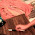## Thursday, April 5, 2012

### Problem 739: Triangle, Double Angle, External Angle Bisector

Geometry Problem
Level: Mathematics Education, High School, Honors Geometry, College.

Click the figure below to see the problem 739 details.1.http://img580.imageshack.us/img580/1161/problem739.png

Extend BC to E such that BE=BA ( see sketch)
Note that ∠ (ABD)= ∠ (EBD)….. ( vertical angles and bisector)
So ∆ (ABD)= ∆EBD…. (Case SAS) => ∠ (BED)= ∠ (BAD)= alpha
In Triangle ECD, external angle ∠ACE= 2alpha= ∠ (CED)+ ∠ (CDE) => ∠CDE= alpha
Triangle ECD is isosceles => CD=BC+BE=BC+AB

2.Locate E on CD such that CE = CB
Let F be any point on extn of AB
∠CBE = ∠CEB = α
∠D =∠ FBD - ∠A = 3α/2 - α = α/2
∠EBD = ∠AEB - ∠D = α - α/2 = α/2
∆BCE, ∆ABE, & ∆BED are all isosceles.
Hence CD = CE + ED = BC + BE = BC + AB

3.let x = AB. Then BC = x/(2cosα).
Then AB + BC = x(1 + 1/(2cosα)).
Now consider triangle BCD. By sine law, CD = ((sin(3α/2))*(x/(2cosα)))/sin(α/2).
Hence it is equivalent to prove (sin(3α/2)/sin(α/2) = 2cosα + 1
By considering the double angle formula, 2cosα + 1 = 3 - 4(sin(α/2))^2
The proof is then obvious by the triple angle formula.

4.Extend AB from B till E so that BE = BC. Draw ED and EC.
The triangle BCE is isosceles then the bisector BD is perpendicular to EC.
Therefore the triangle CED is isosceles, ED = CD.
Let the angle (CBD) = (DBE) = β. Then 2β = 3α.
In the triangle BCD the angle (BDC) = 180 – (180 - 2α) – β = 2α – β; then the angle (EDC) = 2 (BDC) = 4α - 2β = 4α - 3α = α.
Therefore the triangle AED is isosceles. Then AE = AB + BC = ED = CD

5.Let X be the ex-center of ∆ABC opposite the vertex A.
Draw XY ⊥ AB(extended) and XZ ⊥ AD
It is well-known that
the length of the tangent from A to the ex-circle
= AZ = semi-perimeter of ∆ABC
and ∠XDA = ∠YBD - ∠A = 3α/2 - α = α/2
So ∆AXD is isosceles and XZ ⊥ AD
Follows Z is the mid-point of AD
∴ AC + CD = AD = 2AZ = Perimeter of ∆ABC = AC + AB + BC,
Hence CD = AB + BC

6.7.To Rengaraj

Your sketch and the presentation is really nice.
I wonder what software did you use to draw it .

8.I use corelDRAW X5 for sketching, then I export the diagram as JPG to Microsoft word 2010. I have a software called MathType to make efective mathematic typing.

Thaks Peter
Have a nice Day.

9.Picture: http://i1237.photobucket.com/albums/ff480/Evan_Liang/DoubleangleProblem1.jpg
∠BAC=a ∠BCA=2a
Locate F on AC so that BF=AF
∴∠BAC=∠ABF=a (base angles theorem)
∴∠BFC=2a (exterior angles theorem)
∵∠BCA=2a
∴BF=BC (converse of base angles theorem)
locate E on the extended segment of AC so that AE=AB
∴∠BEA=∠EBA=a/2
Goal: Prove CE=FD so then EF=CD=AE+AF=AB+BC
∵∠GBC is the exterior angle of ∠ABC
∴∠GBC=3a
∴∠CBD=3a/2
∵∠EBA=a/2,∠ABF=a
∴∠EBF=a+a/2=3a/2=∠CBD
∴∠EBC=∠FBD
∵BC=BF,∠BFC=∠BCF
∴△CBE≅△FBD
∴CE=DF
∴CD=EF=AE+AF=AB+BC

10.Solution 739 sent by Sumith Peiris, Sri Lanka

Find E on CD such that BE=ED
Now < CBD=3A/2 and so <BDE=<BED=A/2 (A= alpha)

So Tr BCE and Tr ABE are also isoceles, hence CD= CE+ED= BC+AB

Sumith Peiris

Sri Lanka